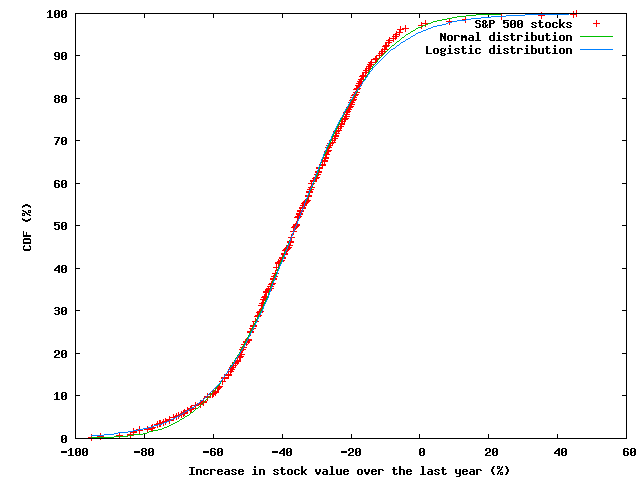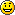# Historical stock data

Is there someplace I can download historical stock data in a machine-friendly format?

I want to test the following hypothesis: investing in 10 stocks chosen randomly from the S&P 500 Index has a similar return to the S&P 500 Index as a whole.

I'm interested in long-term effects, so one quote per stock per year would be sufficient. Some kind of server that I could programmatically query for the closing price of a ticker symbol on a certain date would be awesome.

### 11 comments to Historical stock data

•stenz

Go to Yahoo quotes, then go to any quote, then go down to where it says “download to spreadsheet” and then look at the link.

You can adjust the parameters for dates and ticker, amongst other things.

I’ve used that for data feed stuff many times.

•stenz

Also note that you can put in today’s date for the more recent date, and then a date like 50 years previous (although I would go more 10-20), and it will get that much data if it can, otherwise it errs on giving you what it does have.

•spidurman

If you just go to the historical quotes from another page on that stock, you’ll see what the maximum range is for that security.

•Daniel Stutzbach

Neat. I found a Python script that someone else wrote to download all of the data (from Yahoo) for a list of ticker symbols.

•neuromancerzss

Isn’t your hypothesis here just “random sampling is a valid polling tactic”? If you chose 500 random samples from the S&P, they’d perform exactly like the S&P. If you drop 1, it’ll just be nearly exactly as you’ve introduced some randomness. Induct down to 10 and you’ve just got a higher error rate as the random group clusters towards one end of the profitability scale (that is only known in hindsight of course), but you haven’t changed the core activity of random sampling.

•freelikebeer

I think it is more precisely a central limit theorem kind of thing [law of large numbers, maybe].

•Daniel Stutzbach

See my other response.

•Daniel Stutzbach

You’re right that this is a sampling problem.

Another way of phrasing my question is this: how many stocks do I need to sample in order to get the error rate satisfactorily low?

I suppose another way I could tackle the problem would be to study the distribution of changes in value of the S&P 500 stocks.

Here’s a plot I just made of the percentage change in the S&P stocks from a year ago today, along with fits to normal and logistic distributions:That’s close enough to a normal distribution for me. Standard deviation is around 20 percentage points, so the average of 10 stocks should have a standard deviation of 20/sqrt(10) = 6.3 percentage points.

•freelikebeer

Tweak

The standard deviation of the mean of your ten stocks from the mean of the S&P 500 will be about 6 percentage points. The actual standard deviation of your outcomes will be sqrt(20^2 + 6^2) [which, in and of itself isn’t quite right, but closer], which is like 21%.

•Daniel Stutzbach

Re: Tweak

The 20 percentage points I computed was the variance of all the S&P stocks around the mean of the S&P stocks over one year. The variance of the mean between one year and another is a completely different ball of wax. The actual standard deviation of the outcomes would be sqrt(X^2 + 6^2) but I haven’t tried to estimate X.

•freelikebeer

Re: Tweak

I think we agree. There is a mean and standard deviation for the underlying distro [all S&P500 stocks], and then there is a standard deviation for your sample means with respect to the S&P500 mean, governed by the Central Limit Theorem. The sum [L2-norm] of those two standard deviations gives your the standard deviation of your sample outcomes [but not exactly, because, I think there is some consideration because the values aren’t independent].

As far as year-over-year, if you are choosing randomly, I think that your sample distribution will be stationary with respect to the S&P500 distro. Actually predicting the S&P500 movements, well, good luck.